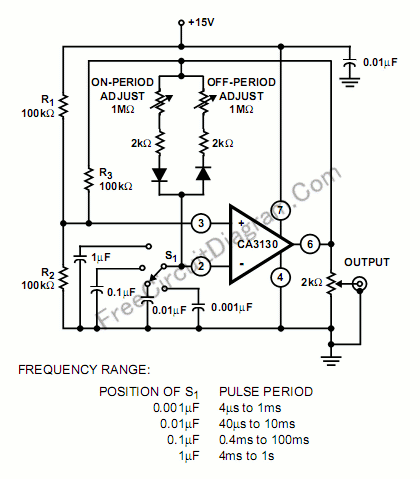# Pulse Generator (Oscillator) With Adjustable On-Off Period Using CA3130 Op-AmpOne of the interesting features of CA3130 op-amp  is its high input resistance, because using such high  input resistance, we can build an astable multivibrator with high R/C ratios. The figure below shows the circuit’s schematic diagram, you can see the variable resistors to adjust the “on” and “off” periods independently.Resistors R1and R2 are used to bias the CA3130 to the mid-point of the supply-voltage and R3 is the feedback resistor. A voltage divider consist of R1 and R2 is used to provide mid-point of supply voltage for the CA3130 biasing, and a feedback loop is provided by R3. S1 is provided to select the pulse repetition rate without affecting the duty factor (on/[on+off] period ratio) set by the variable resistors. [Circuit schematic diagram source: Harris Semiconductor Application Notes]